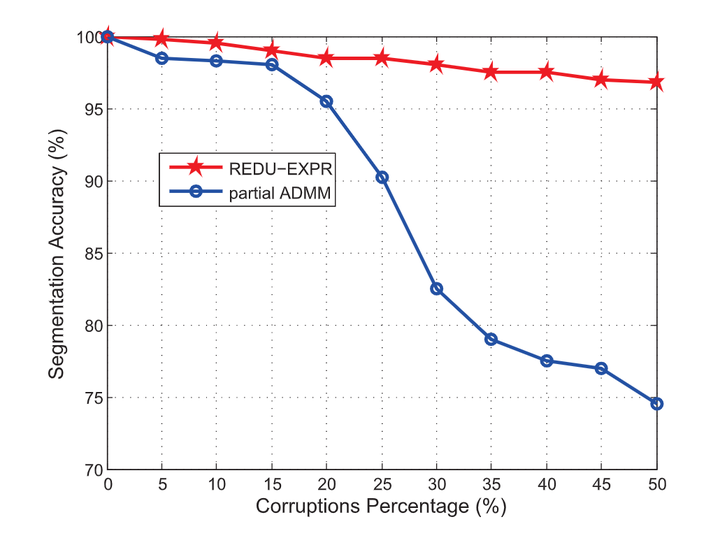# Relations among Some Low Rank Subspace Recovery Models### Abstract

Recovering intrinsic low-dimensional subspaces from data distributed on them is a key preprocessing step to many applications. In recent years, a lot of work has modeled subspace recovery as low-rank minimization problems. We find that some representative models, such as robust principal component analysis (R-PCA), robust low-rank representation (R-LRR), and robust latent low-rank representation (R-LatLRR), are actually deeply connected. More specifically, we discover that once a solution to one of the models is obtained, we can obtain the solutions to other models in closed-form formulations. Since R-PCA is the simplest, our discovery makes it the center of low-rank subspace recovery models. Our work has two important implications. First, R-PCA has a solid theoretical foundation. Under certain conditions, we could find globally optimal solutions to these low-rank models at an overwhelming probability, although these models are nonconvex. Second, we can obtain significantly faster algorithms for these models by solving R-PCA first. The computation cost can be further cut by applying low-complexity randomized algorithms, for example, our novel filtering algorithm, to R-PCA. Although for the moment the formal proof of our filtering algorithm is not yet available, experiments verify the advantages of our algorithm over other state-of-the-art methods based on the alternating direction method.

Type
Publication
Neural Computation